#### Super Conductivity-Basic Phenomenon, Physics tutorial

Introduction:

Superconductivity was first introduced and so named by Kamerlingh Onnes in the year 1911. In the course of an examination of the electrical resistance of different metals at liquid helium temperatures, he noticed that the resistance of a sample of mercury dropped from 0.08 Ω at around 4 K to less than 3 x l0-6 Ω over a temperature interval of around 0.01K. Subsequent attempts illustrated that the width of the transition area in a specific specimen depends on a number of factors, like the purity and metallurgical history and can be as sharp as one mill degree or spread over some degrees. Whereas the breadth of the transition might rise if the sample is metallurgically imperfect, the extraordinary smallness of the resistance in the superconducting state appears to hold for all the superconductors. Therefore, the first feature property of a superconductor is that its electrical resistance, for all the practical objectives, is zero, beneath a well-defined temperature Tc, termed as the critical, or transition temperature. Therefore, the conductivity in this range of temperature is infinite; therefore the nomenclature of superconductivity.

The figure below illustrates how the electrical resistivity in a superconductor becomes immeasurably small at the transition temperature. The figure as well contrasts the behavior of a normal metal for which at extremely low temperatures, the remanent resistivity is the feature of residual impurities. The resistance of a superconductor is believed to be zero instead of just very small.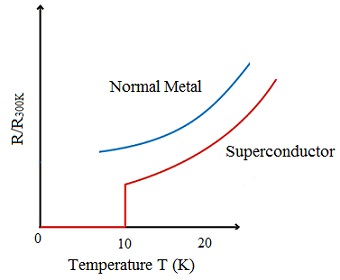Definition:

The Superconductivity is a phenomenon on which the electrical resistivity of metals or alloys drop to zero (that is, infinite conductivity) whenever cooled to its critical temperature.

Empirical criteria:

There are found to be a number of regularities in the appearance of the superconductivity, the principal of which are given:

1) Superconductivity has been noticed only for such metallic substances for which the number of valence electrons 'Z' lies between 2 and 8.

2) In all the cases comprising transition metals, the variation of Tc with the number of valence electrons illustrates sharp maxima for Z = 3, 5 and 7, as illustrated in the figure given below: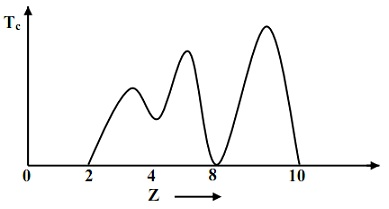3) A rather striking correlation (that is, a straight line graph) exists between the 3 and Z2 for elements all along given rows of the periodic table.

4) For a given value of 'Z', some crystal structures seem more favorable than the others. For illustration, β-tungsten and α-manganese structure are conductive to the phenomenon of the superconductivity.

5) Ferromagnetic and ferroelectric ordering is found to slow down the superconductivity.

6) Tc rises by a high power of the atomic volume and inversely as the atomic mass.

7) Superconductivity takes place in materials having high normal resistivity. The condition n p > 106 is a good criterion for the existence of the superconductivity, here 'n' is the number of valence electrons per c.c. and 'p' is the resistivity in electrostatic units at 20°C.

Such empirical rules have played a significant role in the discovery of latest superconductors.

Transition Temperature:

The temperature at which the normal metal passes to the superconducting state is termed as the transition temperature, Tc. The transition temperature is usually influenced by the application of the pressure although no specific regularity in the behavior has been found. The value of Tc for most of the metals lies beneath 4K; example: for Al, it is 1.20 K. For C-15 structure (example: V2 Hf), it is 10K; for B-1 structure (example: NbN), it is close to 13 K, while NbZr and NbT1 [BCC (A-2) structure] contain the values of Tc as 11.0 and 10.0 K correspondingly. For A-15 structure, the highest Tc = 23.2 K has been noticed in NB3Ge.

Energy Gap:

Experiments have illustrated that in superconductors, for temperatures in the vicinity of absolute zero, a forbidden energy gap just over the Fermi level is noticed. The first part of figure illustrated below represents the conduction band in the normal state, whereas the second part shows an energy gap equivalent to 2Δ at the Fermi level in the superconducting state. Therefore, the Fermi level in a superconductor is midway between the ground state and the first excited state in such a way that each lies an energy distance = Δ away from the Fermi level. Electrons in the excited states above the gap behave as the normal electrons. At absolute zero, there are no electrons over the gap. 2Δ is usually of the order of 10-4 eV.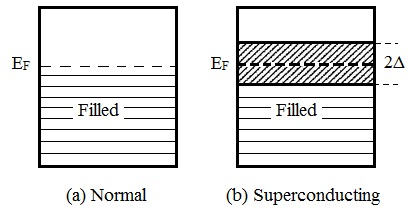Δ is found to be the function of temperature 'T'. Therefore, (T) symbolizes energy gap at temperature T. The figure represented below illustrates reduced values of observed energy gap (T)/Δ (0) as a function of the reduced temperature T/Tc. Elementary theory expects that:

Δ(T)/Δ(0) = 1.74 (1 - T/TC)1/2

We notice that the energy gap reduces continuously to zero as the temperature is raised to Tc. numerically; the experiments show that for most of the metals. The transition from the superconducting state to the normal state is noticed to be a second-order phase transition. In such a transition, there is no latent heat; however there is a discontinuity in the heat capacity.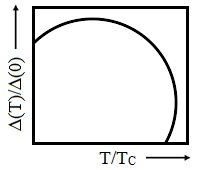Properties Dependent on Energy Gap:

1) Microwave and Infrared Absorptions:

The reaction of a metal to electromagnetic radiation is found out by the frequency dependent conductivity. This in turn based on the available methods for energy absorption via the conduction electrons at given frequency. As the electronic excitation spectrum in the superconducting state is characterized via an energy gap 2Δ, one would anticipate the AC conductivity to differ considerably from its normal state form at frequencies small compared with [(2Δ)/(h/2π)], and to be effectively similar in the superconducting and normal states at frequencies large compared with [(2Δ)/(h/2π)]. The value of [(2Δ)/(h/2π)], is usually in the range between microwave and infrared frequencies. In the superconducting state, AC behavior is observed that is indistinguishable from that in the normal state at the optical frequencies. Deviations from the normal state behavior first appear in the infrared, and only at microwave frequencies does AC behavior completely displaying the lack of electronic absorption feature of an energy gap becomes fully developed.

2) Density of States:

The three portions of the figure shown below provide a highly exaggerated picture of the difference between the spectrum and occupancy of states in the normal metal and those in a superconductor. Part first considers the density of states at T = 0 in the absence of the superconductivity (that is, can be arranged via applying an appropriate magnetic field). The superconducting ground state for zero temperature is pictured in the second part of the figure. This represents a zero density of states for energies within ± Δ(0) on either side of the Fermi energy, and a piling up of the displaced states on either side of the gap. At T - 0, no electrons are excited to the higher states. Third part of the figure assumes the consequences of a finite temperature less than Tc. The superconducting energy gap Δ(0) is now smaller than Δ(0). The fractions of number of electrons are in states above EF + ΔT leaving behind some unoccupied states beneath EF - ΔT. Lastly, the gap reduces to zero if T reaches Tc and the corresponding density of states is the one depicted in the first part.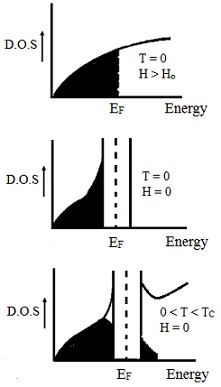3) Specific Heat:

There is no heat of transformation related by the superconducting-normal transition in a metal, however there is an anomaly in the electronic component of the specific heat. An illustration of this is described in the figure shown below. The discontinuity in the specific heat reflects the second-order transition from a relatively disordered (that is, normal) state to a more highly ordered (that is, superconducting) state of lower entropy. At low temperatures, the specific heat of a normal metal consists of the form:

Cn = AT + BT3

Here, the linear word is due to the electronic excitations and the cubic term is due to the lattice vibrations. Beneath the superconducting critical temperature, this behavior is considerably altered. As the temperature falls beneath Tc, the specific heat jumps to a higher value and then slowly reduces, ultimately falling well beneath the value one would anticipate for a normal metal. By applying a magnetic field to drive the metal to the normal state, one can compare the specific heats of the superconducting and normal states beneath the critical temperature.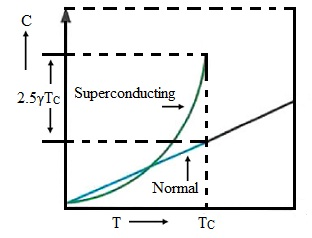Such an analysis discloses that in the superconducting state, the linear electronic contribution to the specific heat is substituted by term that disappears much more rapidly at very low temperatures, having dominant low-temperature behavior of the form exp(-Δ/kBT). This is the feature thermal behavior of a system whose excited levels are separated from the ground state by energy 2Δ, therefore, the net specific heat of the superconducting state is:

CS = AT3CSS

Here, CSS/γT = a exp (-b (TC/t))

Here, 'γT' is the low-temperature electronic specific heat of the normal state (achieved by applying appropriate magnetic field), and a ≈ 9 and b ≈ 1.5. Such parameters are themselves weakly temperature dependent. In the above figure the size of the discontinuity in specific heat at T = Tc is 2.5 in units of γTC. The exponential decrease in specific heat below Tc can be interpreted as follows. Since, of the energy gap, the number of electrons excited across the gap is given roughly via a Boltzmann factor, exp (-Δ/kBT). Therefore, the heat capacity differs exponentially by temperature.

4) Acoustic Attenuation:

If a sound wave propagates via a metal, the microscopic electric fields due to the displacement of the ions can pass on energy to electron close to the Fermi level, thus eradicating energy from the wave. This is deduced by the attenuation coefficient, 'α' of acoustic waves. The ratio of 'α' for superconducting and normal state is represented by:

as/an = 2/[1 + exp(Δ/kBT)]

At low temperatures:

as/an = 2 exp (-Δ/kBT)

The exponential decay ratio is illustrated in the figure shown below.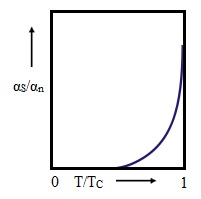5) Thermal Conductivity:

In normal metals, the heat current is mainly carried through the conduction electrons and at low temperatures, the electronic contribution to the thermal conductivity Ken is represented by the Wiedemann-Franz law. In a superconductor, though, the electron pairs encompass zero energy so they can't contribute to energy transport and therefore to the heat current (however being charged, they can still contribute to the electric current). Therefore, the electronic contribution to the heat current based on the number of normal electrons and similar to the electronic specific heat represented by the equation CSS/γT = a exp (-b (TC/t)), we have the ratio of superconducting to normal phase conductivities as:

kss/ksn ≈ exp (-Δ/kBT)

This is described in the figure shown below. If T << TC, Kss → 0 and the only thermal current will be taken out by the phonons (as in insulator). Under appropriate conditions, kss/ksn might be very large (≈ 108) and this property can be employed to make a heat switch, the heat flow being controlled through a magnet. The phonon contribution to thermal conduction will in reality rise in the superconducting state as the scattering of phonons through electrons is decreased by the formation of pairs. In extreme conditions, if ksn is made small through the introduction of impurities, the rise in the phonon contribution to the thermal conductivity beneath TC might outweigh the reduction in the electronic contribution in such a way that the total conductivity rises in the superconducting state. To accomplish this condition, an impurity of similar mass however different valence, that will reduce ksn without greatly influencing phonon transport, must be employed. An illustration is Bi in Pb.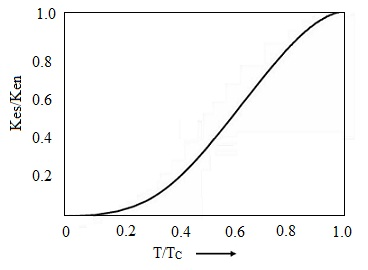Tutorsglobe: A way to secure high grade in your curriculum (Online Tutoring)